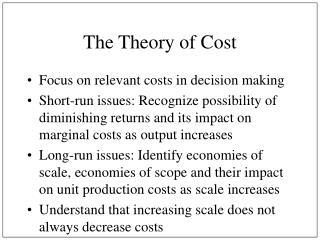Download PresentationThe Theory of Cost

The Theory of Cost - PowerPoint PPT Presentation

The Theory of Cost. Focus on relevant costs in decision making Short-run issues: Recognize possibility of diminishing returns and its impact on marginal costs as output increasesI am the owner, or an agent authorized to act on behalf of the owner, of the copyrighted work described.
Download PresentationThe Theory of Cost

Download Policy: Content on the Website is provided to you AS IS for your information and personal use and may not be sold / licensed / shared on other websites without getting consent from its author.While downloading, if for some reason you are not able to download a presentation, the publisher may have deleted the file from their server.

- - - - - - - - - - - - - - - - - - - - - - - - - - E N D - - - - - - - - - - - - - - - - - - - - - - - - - -
Presentation Transcript
1. The Theory of Cost • Focus on relevant costs in decision making • Short-run issues: Recognize possibility of diminishing returns and its impact on marginal costs as output increases • Long-run issues: Identify economies of scale, economies of scope and their impact on unit production costs as scale increases • Understand that increasing scale does not always decrease costs

2. The Importance of Cost • One of two major factors in profit maximizing decision • What is the other? • Increase sales by \$1, what’s the impact on profit? • Decrease cost by \$1, what’s the impact on profit?

3. Nature of Costs • Historical v. replacement • Opportunity v. out-of-pocket • Sunk v. incremental • Explicit v. implicit • Short-run v. long-run • Fixed v. variable • Economic v. accounting

4. Relevant Costs • Depreciation: Accounting concept often has little relationship with the actual loss of value. • Inventory: Accounting concept based on acquisition cost. • Unutilized facilities: Empty space may appear to have no cost. • Profitability measures: Accounting v. economic

5. Graphing Costs • TC, TFC, TVC • ATC, AFC, AVC • MC • See Figure 9.3, p. 330

6. Relationship between Production and Cost • Production is a key determinant of cost • AVC = TVC/Q = wL/Q = w(L/Q) = w(1/APL) • MC = dTVC/dQ = d(wL)/dQ = w(dL/dQ) + L(dw/dQ) = w(1/MPL)

7. Long-Run Cost Curves • The long run is the planning horizon • We manage the future • All inputs are variable in LR • LAC often referred to as the envelope curve • Refer to Figure 9.4, p. 332

8. Economies of Scale • Output is growing proportionately faster than input use • LAC is downward sloping • Reasons for economies of scale

9. Diseconomies of Scale • Output is growing proportionately slower than input use • LAC is upward sloping • Reasons for diseconomies of scale

10. Learning Curves • Depicts the declining AC over time due to experience in production • Algebraically: C = aQb; where b is negative and represents the rate that input costs decline over time • log C = log a + b log Q

11. Cost-Volume-Profit Analysis • Break-even analysis • Assuming constant prices and constant AVC • Operating leverage • importance of FC in the firm’s operations • examines change in operating profit due to a change in sales volume • important concept--DOL or sales elasticity of operating profit

12. Typical Cost Functions • TC = a + bQ - cQ2 + dQ3 • TFC = a • TVC = bQ - cQ2 + dQ3 • ATC = a/Q + b - cQ + dQ2 • AFC = a/Q • AVC = b - cQ + dQ2 • MC = b - 2cQ + 3dQ2

13. Alternative Cost Functions • Straight-line cost functions • TC = a + bQ • AC = a/Q + b; AVC = MC = b • Increasing at an increasing rate • TC = a + bQ + cQ2 • AC = a/Q + b + cQ; AVC = b + cQ; MC = b + 2cQ • Graphical presentation